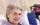One-third

A one-third of unknown number is equal to five times as great as the difference of the same unknown number and number 28.
Determine the unknown number.

Result

x =  30

Solution:

x/3=5(x-28)

14x = 420

x = 30

Calculated by our simple equation calculator.

Leave us a comment of this math problem and its solution (i.e. if it is still somewhat unclear...):Be the first to comment!To solve this verbal math problem are needed these knowledge from mathematics:

Do you have a linear equation or system of equations and looking for its solution? Or do you have quadratic equation?

Next similar math problems:

1. NormThree workers planted 3555 seedlings of tomatoes in one dey. First worked at the standard norm, the second planted 120 seedlings more and the third 135 seedlings more than the first worker. How many seedlings were standard norm?
2. Unknown number 11That number increased by three equals three times itself?
3. Unknown numberIdentify unknown number which 1/5 is 40 greater than one tenth of that number.
4. Find xSolve: if 2(x-1)=14, then x= (solve an equation with one unknown)
5. Arithmetic averageThe arithmetic mean of the five numbers is exactly 8. The sum of these four numbers is 30. What is the fifth number?
6. Dropped sheetsThree consecutive sheets dropped from the book. The sum of the numbers on the pages of the dropped sheets is 273. What number has the last page of the dropped sheets?
7. EquationSolve the equation: 1/2-2/8 = 1/10; Write the result as a decimal number.
8. Forest nurseryIn the forest nursery after winter, they found that 1/10 stems died out of them. For them, they land 193 new spruces. How many spruces are in the forest nursery?
9. Equation?
10. Simple equationSolve for x: 3(x + 2) = x - 18
11. CakesGrandmother baked cakes. Half of its was poppy, quarter with plum jam and 16 cheesecakes. How many cakes she baked in total?
12. Simple equation 9Solve the following equation: -8y+5=-9y+9
13. Simple equation 8Solve the following equation: 36=-(1+7x)-6(-7-x)
14. Equation 29Solve next equation: 2 ( 2x + 3 ) = 8 ( 1 - x) -5 ( x -2 )
15. Simple equationSolve the following simple equation: 2. (4x + 3) = 2-5. (1-x)
16. Negative in equation2x + 3 + 7x = – 24, what is the value of x?
17. FamilyMom is 39 years old. Karl is eight years and Paul 4 years. For many years mother would old just like two children together?